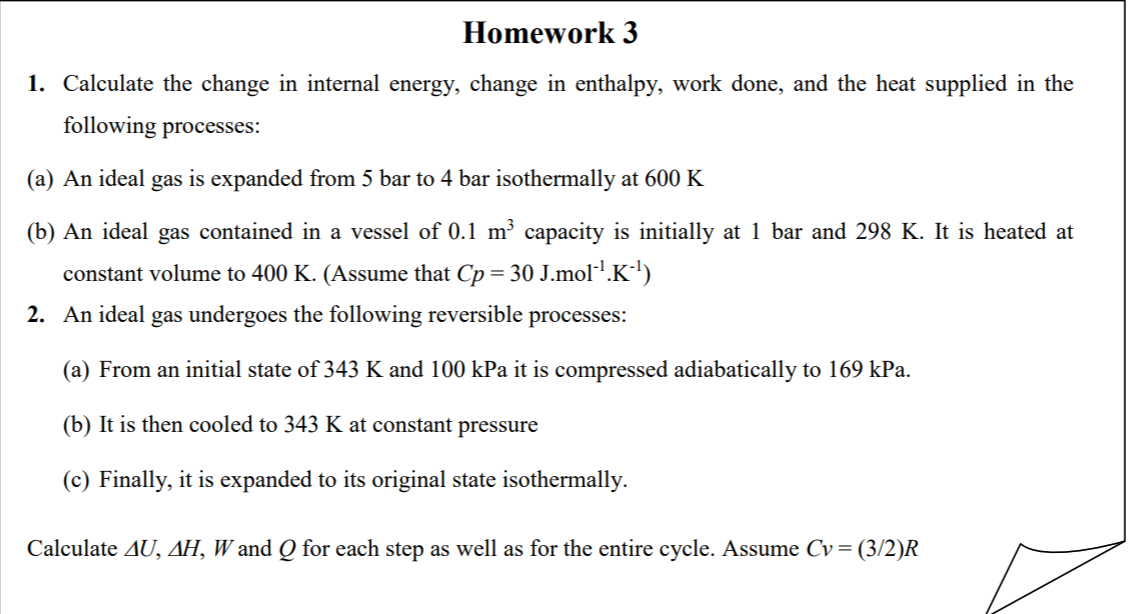# 1. Calculate the change in internal energy, change in enthalpy, work done, and the heat supplied in the following processes: (a) An ideal gas is expanded from 5 bar to 4 bar isothermally at 600 K (b) An ideal gas contained in a vessel of 0.1 m² capacity is initially at 1 bar and 298 K. It is heated at constant volume to 400 K. (Assume that Cp = 30 J.mol-!.K-4) 2. An ideal gas undergoes the following reversible processes: (a) From an initial state of 343 K and 100 kPa it is compressed adiabatically to 169 kPa. (b) It is then cooled to 343 K at constant pressure (c) Finally, it is expanded to its original state isothermally. Calculate AU, AH, W and Q for each step as well as for the entire cycle. Assume Cv = (3/2)R

Question-AnswerCategory: Chemical Thermodynamics1. Calculate the change in internal energy, change in enthalpy, work done, and the heat supplied in the following processes: (a) An ideal gas is expanded from 5 bar to 4 bar isothermally at 600 K (b) An ideal gas contained in a vessel of 0.1 m² capacity is initially at 1 bar and 298 K. It is heated at constant volume to 400 K. (Assume that Cp = 30 J.mol-!.K-4) 2. An ideal gas undergoes the following reversible processes: (a) From an initial state of 343 K and 100 kPa it is compressed adiabatically to 169 kPa. (b) It is then cooled to 343 K at constant pressure (c) Finally, it is expanded to its original state isothermally. Calculate AU, AH, W and Q for each step as well as for the entire cycle. Assume Cv = (3/2)R

1. Calculate the change in internal energy, change in enthalpy, work done, and the heat supplied in the following processes: (a) An ideal gas is expanded from 5 bar to 4 bar isothermally at 600 K (b) An ideal gas contained in a vessel of 0.1 m² capacity is initially at 1 bar and 298 K. It is heated at constant volume to 400 K. (Assume that Cp = 30 J.mol-!.K-4) 2. An ideal gas undergoes the following reversible processes: (a) From an initial state of 343 K and 100 kPa it is compressed adiabatically to 169 kPa. (b) It is then cooled to 343 K at constant pressure (c) Finally, it is expanded to its original state isothermally. Calculate AU, AH, W and Q for each step as well as for the entire cycle. Assume Cv = (3/2)R